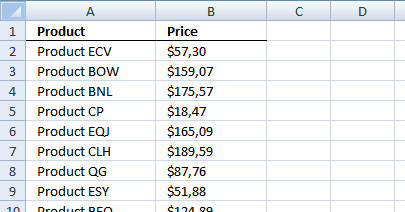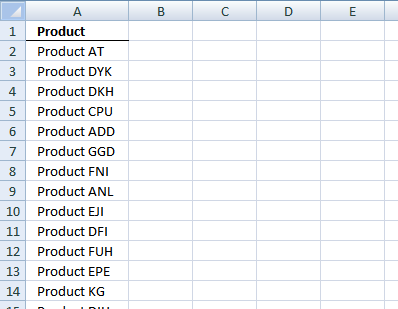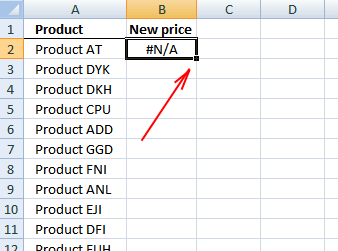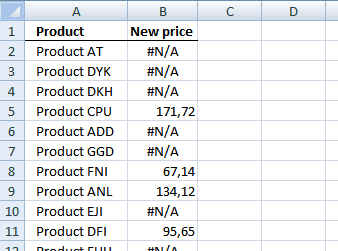Author: Oscar Cronquist Article last updated on December 08, 2018

### Overview

Updating a list using copy/paste is a boring task. This blog article describes how to update values in a price list with new values.

Sheet 1 is the old price list. It contains 5000 products and amounts.Sheet2 is the new price list. It contains 2000 random products from the old price list with new prices. (There are no new products)### How to create a new list with the latest prices.Now let us find out if a new price exists.

### Sheet3, formula in B2:

=INDEX(Sheet2!\$B\$2:\$B\$2001, MATCH(A2, Sheet2!\$A\$2:\$A\$2001, 0)) + ENTER.Double press with left mouse button on lower right corner of cell B2.The formula is copied down to last adjacent product.

### Sheet3, formula in C2:

=IF(ISERROR(MATCH(A2, Sheet2!\$A\$2:\$A\$2001, 0)), INDEX(Sheet1!\$B\$2:\$B\$5000, MATCH(A2, Sheet1!\$A\$2:\$A\$5000, 0)), INDEX(Sheet2!\$B\$2:\$B\$2001, MATCH(A2, Sheet2!\$A\$2:\$A\$2001, 0))) + ENTER.

Double press with left mouse button on lower right corner of cell C2 to copy the formula as far down as needed.I created column B to make sure the values are the same as in sheet2 and to make it easier to understand the formula in cell C2.

### Explaining the formula in cell C2

Step 1 - Find out if a new price exists

=IF(ISERROR(MATCH(A2, Sheet2!\$A\$2:\$A\$2001, 0)), INDEX(Sheet1!\$B\$2:\$B\$5000, MATCH(A2, Sheet1!\$A\$2:\$A\$5000, 0)), INDEX(Sheet2!\$B\$2:\$B\$2001, MATCH(A2, Sheet2!\$A\$2:\$A\$2001, 0)))

Match returns the relative position of an item in (Sheet2!\$A\$2:\$A\$2001) that matches a specified value (A2).

MATCH(A2, Sheet2!\$A\$2:\$A\$2001, 0) returns #N/A. This means "Product AT" can´t be found in sheet2.

ISERROR(MATCH(A2, Sheet2!\$A\$2:\$A\$2001, 0)) returns TRUE.

Step 2 - Identify sheet and price

The formula returned TRUE in cell C2. This means there is no new price. Let´s find the old price in sheet1 instead.

=IF(ISERROR(MATCH(A2, Sheet2!\$A\$2:\$A\$2001, 0)), Formula if TRUE, Formula if FALSE)

=IF(ISERROR(MATCH(A2, Sheet2!\$A\$2:\$A\$2001, 0)), INDEX(Sheet1!\$B\$2:\$B\$5000, MATCH(A2, Sheet1!\$A\$2:\$A\$5000, 0)), INDEX(Sheet2!\$B\$2:\$B\$2001, MATCH(A2, Sheet2!\$A\$2:\$A\$2001, 0)))

INDEX(Sheet1!\$B\$2:\$B\$5000, MATCH(A2, Sheet1!\$A\$2:\$A\$5000, 0))

MATCH(A2, Sheet1!\$A\$2:\$A\$5000, 0) returns 1. "Product AT" is found on the first row on sheet1.

INDEX(Sheet1!\$B\$2:\$B\$5000, 1) returns \$43,90.

If formula in step 1 had returned False

If the formula had returned False in cell C2, we would need to look for the new price in sheet2.

=IF(ISERROR(MATCH(A2, Sheet2!\$A\$2:\$A\$2001, 0)), Formula if TRUE, Formula if FALSE)

=IF(ISERROR(MATCH(A2, Sheet2!\$A\$2:\$A\$2001, 0)), INDEX(Sheet1!\$B\$2:\$B\$5000, MATCH(A2, Sheet1!\$A\$2:\$A\$5000, 0)), INDEX(Sheet2!\$B\$2:\$B\$2001, MATCH(A2, Sheet2!\$A\$2:\$A\$2001, 0)))

INDEX(Sheet2!\$B\$2:\$B\$2001, MATCH(A2, Sheet2!\$A\$2:\$A\$2001, 0))

Get excel example file
automate_excel_pricelist.zip (300 KB)